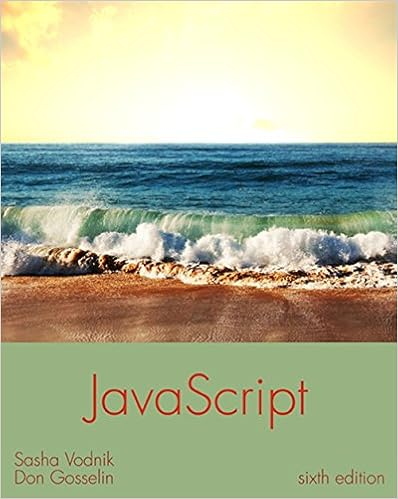# Debug09 02 program gets two numbers and computes

• Homework Help
• 46
• 97% (175) 169 out of 175 people found this document helpful

This preview shows page 40 - 44 out of 46 pages.

##### We have textbook solutions for you!
The document you are viewing contains questions related to this textbook.The document you are viewing contains questions related to this textbook.
Chapter 2 / Exercise 4
JavaScript: The Web Warrior Series
VodnikExpert Verified
40
##### We have textbook solutions for you!
The document you are viewing contains questions related to this textbook.The document you are viewing contains questions related to this textbook.
Chapter 2 / Exercise 4
JavaScript: The Web Warrior Series
VodnikExpert Verified
Programming Logic and Design, 8eSolutions 9-computeProduct(firstNumber, secondNumber)// firstNumber and secondNumber must be passed to methodstopvoid computeProduct(num first, num second)// Method type is voidDeclarationsnum answeranswer = first * secondoutput first, "times ", second, " is ", answerreturnDEBUG09-03// Program passes an array to a method that reverses// the positions of the valuesstartDeclarationsnum LENGTH = 4num vals[LENGTH] = 33, 55, 77, 99num suboutput "At beginning of main() method..."sub = 0while sub < LENGTH// This is a loop, not a selectionoutput vals[sub]sub = sub + 1endwhile// while loop end with endwhilereverseTheValues(vals)output "At end of main() method.........."sub = 0while sub < LENGTHoutput vals[sub]sub = sub + 1endwhilestopvoid reverseTheValues(num[] values)// parameter needs brackets becasue it represents an arraynum temptemp = valuesvalues = valuesvalues = temp// temp goes to valuestemp = valuesvalues = valuesvalues = tempreturn2.Your downloadable files for Chapter 9 include a file named DEBUG09-04.jpg that contains a flowchart with syntax and/or logical errors. Examine the flowchart and then find and correct all the bugs.Answer:41
Programming Logic and Design, 8eSolutions 9-Game Zone1.In the Game Zone sections of Chapters 6 and 8, you designed the logic for a quiz that contains questions about a topic of your choice. Now, modify the program so it contains an array of five multiple-choice quiz questions related to the topic of your choice. Each question contains four answer choices. Also create a parallel array that holds the correct answer to each question—A, B, C, or D. In turn, pass each question to a method that displays the question and accepts the player’s answer. If the player does not enter a valid answer choice, force the player to reenter the choice. Return theuser’s valid (but not necessarily correct) answer to the main program. After the user’s 42
Programming Logic and Design, 8eSolutions 9-answer is returned to the main program, pass it and the correct answer to a method that determines whether the values are equal and displays an appropriate message. After the user answers all five questions, display the number of correct and incorrect answers that the user chose.Answer: A sample solution followsPseudocode:startDeclarationsnum correctnum xnum SIZE = 5string guesses[SIZE] = “”string QUESTIONS[SIZE] = “What is the closest star to Earth?”, “What is the name of Earth’s moon?”, “How many miles (in trillions) are in a light year?”, “How many moons does Pluto have?”,
•••